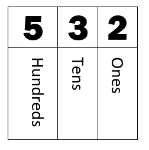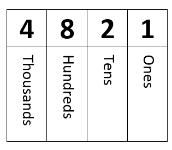# Math Worksheets Land

Math Worksheets For All Ages

# Math Worksheets Land

Math Worksheets For All Ages

# Tens, Hundred, and Thousands Place Worksheets

Understanding what the significance of each integer in a number is a core and fundamental math skill that students will need to master at this level and carry on to all future grades with them. This helps them understand a great deal about basic numeracy and it makes calculating various math operations much easier. When we move on to the concepts of decimal values understanding this topic will be the first step in that direction. This collection of worksheets will have students identifying and actively using place values.

### Aligned Standard: Grade 4 Base Ten - 4.NBT.1

• Answer Keys - These are for all the unlocked materials above.

### Homework Sheets

We start by tackling the number of thousands, hundreds, tens, and ones in various numbers.

• Homework 1 - How many ones you can make from 2 thousand? We move from ones to tens to hundreds to thousands. 3 places to the right.
• Homework 2 - A good example of a problem on this sheet would be: ____ thousands = 80 hundreds.
• Homework 3 - Solve these problems out right. You will need to add a concept of balance.

### Practice Worksheets

Very similar to the homework, but this time we space out more.

• Practice 1 - Complete all the boxes please. You will explore the use of word form and expanded form to balance each of the equations.
• Practice 2 - Fill the empty cells with an equal value to the other side.
• Practice 3 - Another worksheet for you. Take your time with these problems.

### Math Skill Quizzes

I used the most people place value shifts that you will see assessed at all the various levels.

• Quiz 1 - These quiz sheets will make for quick grading and feedback for students.
• Quiz 2 - Find the value of interest and then complement it on the other side of the equals symbol.
• Quiz 3 - This is a bit more challenging. Great to see how far they have come along with this section.

### What Is Meant by the Tens, Hundred, and Thousands Place?

A place value is the worth of an integer within a number. For instance, in the number 4,831, the 4 represents 4,000, the 8 signifies 800, the 3 represents 30, and the 1 stands for 1. If you notice as you move from right to left each value is ten times greater than the previous one. This is why we call the numeracy system that we use the base ten system. The goal here is to understand what value each of the integers found within a number represents.

To understand what tens, hundreds, and thousands of places mean, you must learn about the concept of place values. You can visualize place values as the fingers in your hand, the fingers are called digits, and each finger can be the number 0 – 9. In a number, the value of the digit can be found out by its position. For instance, the number 532 has five 100s, three 10s and two 1s added together to form one number. Let's visualize them further in the table below.If you add these three numbers together (500 + 30 +2), you will again get the answer 532. So, if the above number tells us about the hundreds, tens, and units place of an expression, where will the thousands place come? It will be placed on the leftmost side in a 4-digit number.

Let's take another example of the expression 4821 in the table below.Things to Remember

- Thousands place number has three zeros.
- Hundreds place number has two zeros.
- Tens place number has a single zero.
- One's place digit does not have a zero. So in this case the value is 4,000 + 800 + 20 +1.

Unlock all the answers, worksheets, homework, tests and more!
Save Tons of Time! Make My Life Easier Now

## Thanks and Don't Forget To Tell Your Friends!

I would appreciate everyone letting me know if you find any errors. I'm getting a little older these days and my eyes are going. Please contact me, to let me know. I'll fix it ASAP.